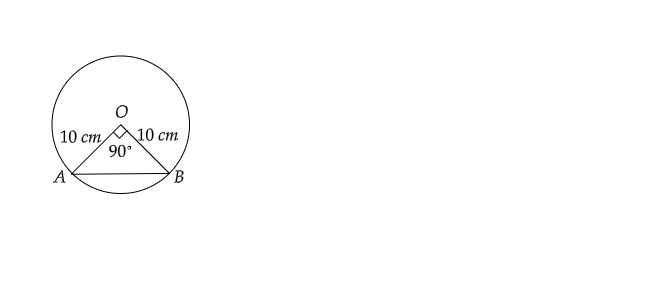# A chord of a circle of radius 10 cm subtends a right angle at the centre. Find the area of the corresponding:(i) minor segment.(ii) major segment.

Given:

A chord of a circle of radius $10\ cm$ subtends a right angle at the center.

To do:

We have to find the area of the corresponding minor sector.

Solution:Let $AB$ be the chord which subtends an angle $90^o$ at the center $O$.

(i) Area of the minor sector$=$Area of the segment $AOB-$Area of $\vartriangle AOB$

$=\frac{\pi\theta}{360^{\circ}}\times OA\times OB-\frac{1}{2}\times OA\times OB$

$=\frac{3.14\times 90^{\circ}}{360^{\circ}}\times 10\times 10-0.5\times 10\times10$

$=78.5-50$

$=28.5\ cm^2$

Therefore, the area of the minor sector is $28.5\ cm^2$.

(ii) As the radius of the circle$=OA=OB=10\ cm$

Angle subtended by the chord $=\angle AOB=\theta=90^{\circ}$

Area of the major sector$=$ Area of the circle$-$Area of the minor sector

$=\pi r^2-\frac{\pi\theta}{360^{\circ}}\times OA\times OB$

$=3.14\times10\times10-3.14\times\frac{90^{\circ}}{360^{\circ}}\times10\times10$

$=314-78.5$

$=235.5\ cm^2$

Therefore, the area of the major sector is $235.5\ cm^2$.

Updated on: 10-Oct-2022

7K+ Views